# Selina Solutions Concise Maths Class 7 Chapter 4: Decimal Fractions (Decimals) Exercise 4A

Selina Solutions Concise Maths Class 7 Chapter 4 Decimal Fractions (Decimals) Exercise 4A explains concepts like converting decimal numbers into fractions and vice versa and decimal places. The solutions are available in PDF format, which helps students ace the exam with good marks. Solving the exercise wise problems help students enhance their analytical thinking abilities, which are important from the exam point of view. Students can download Selina Solutions Concise Maths Class 7 Chapter 4 Decimal Fractions (Decimals) Exercise 4A PDF, from the links which are given below.

## Selina Solutions Concise Maths Class 7 Chapter 4: Decimal Fractions (Decimals) Exercise 4A Download PDF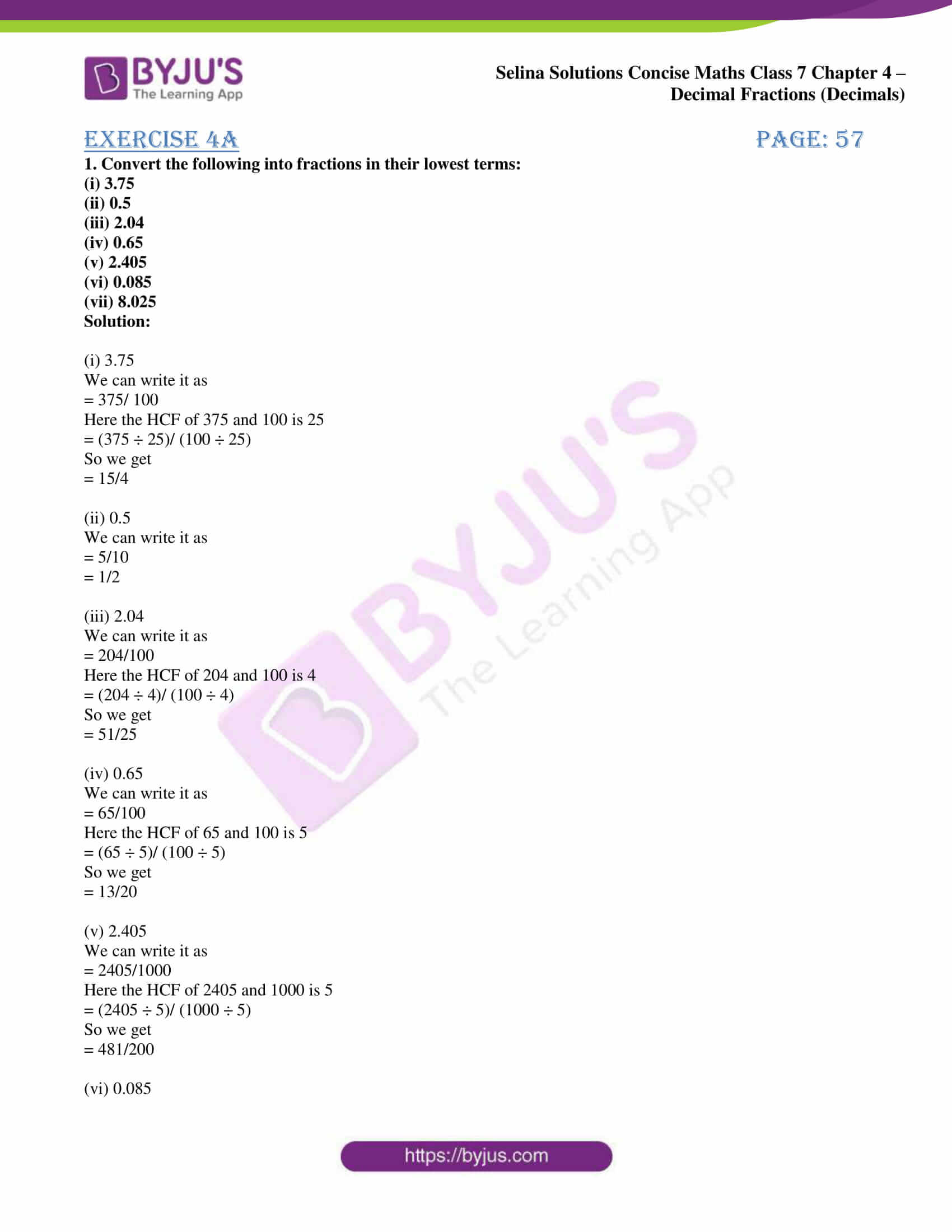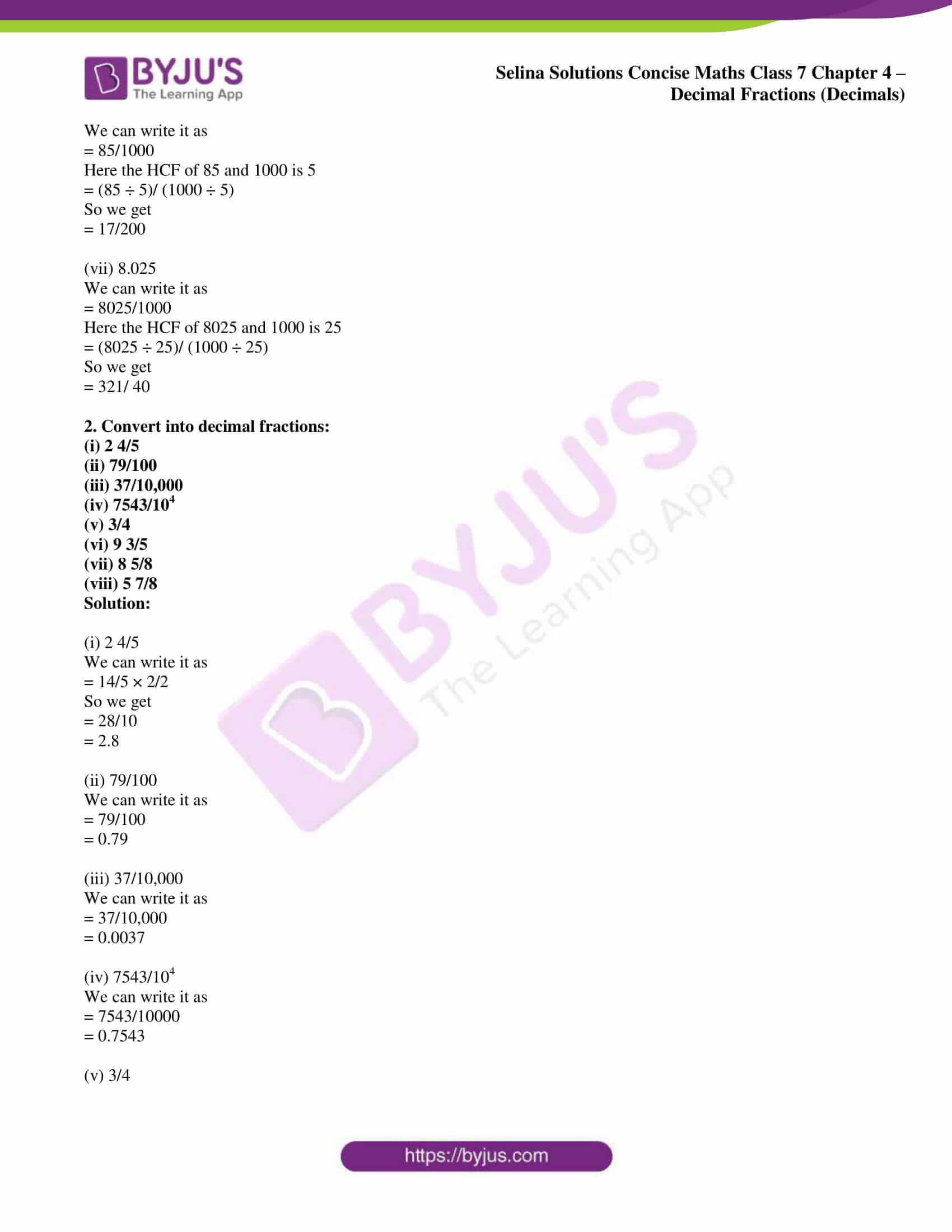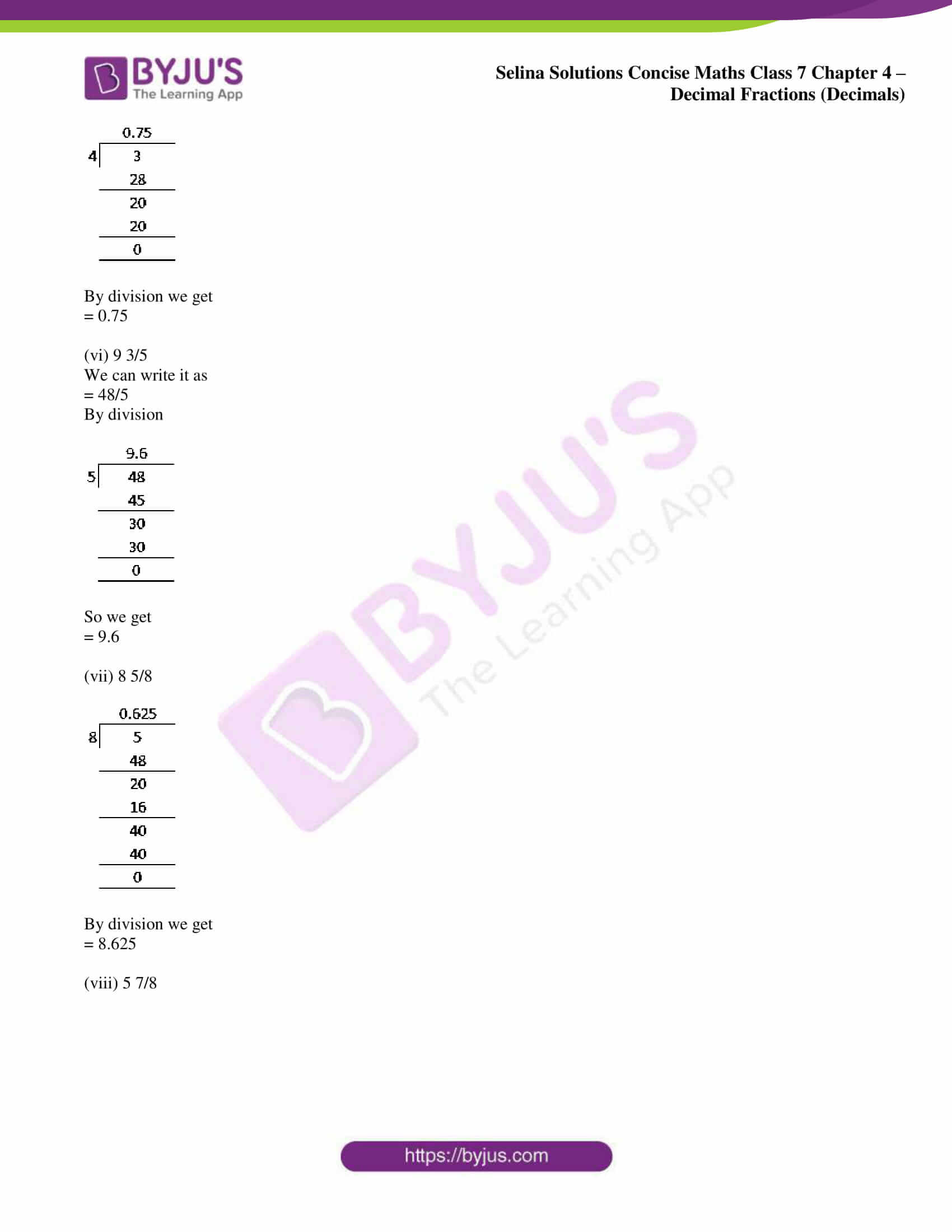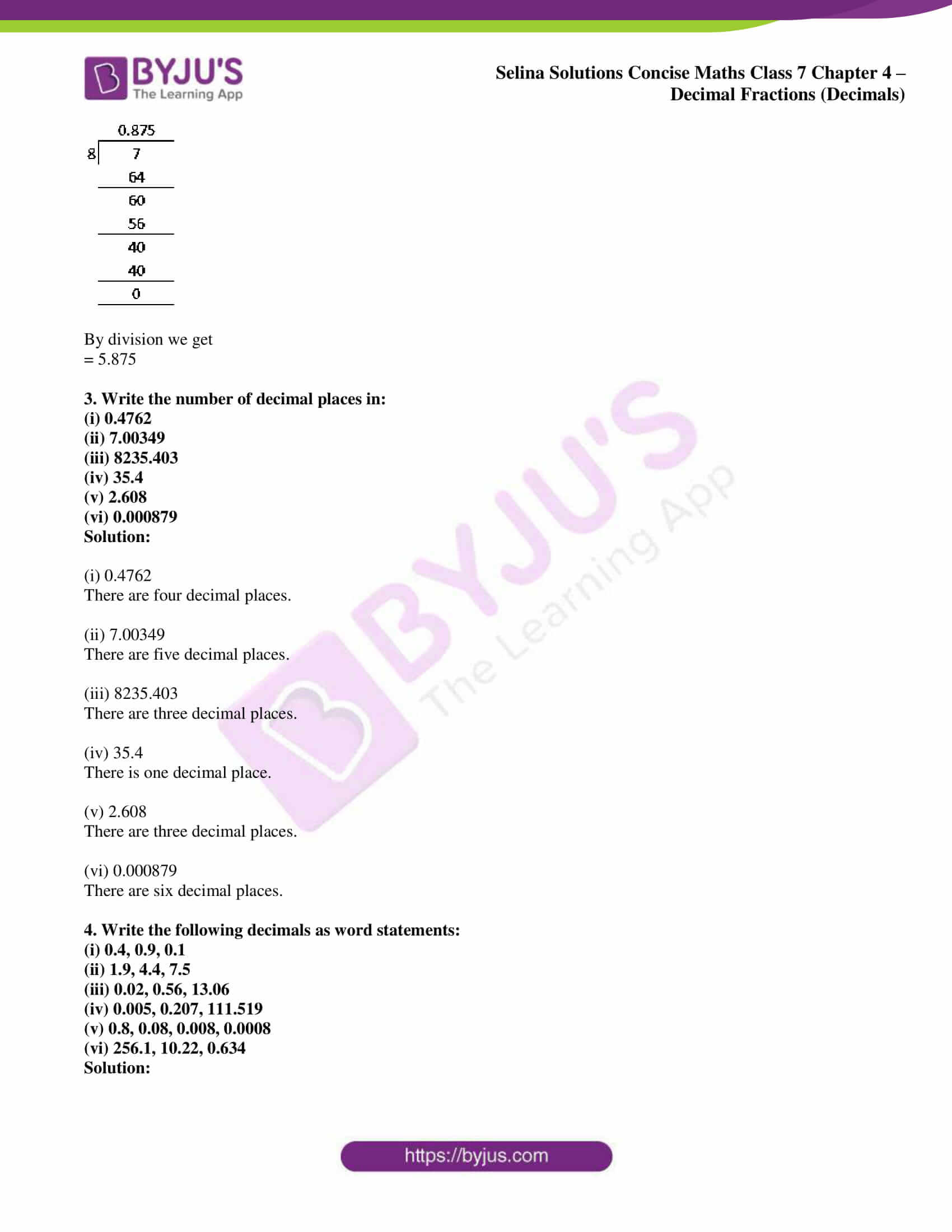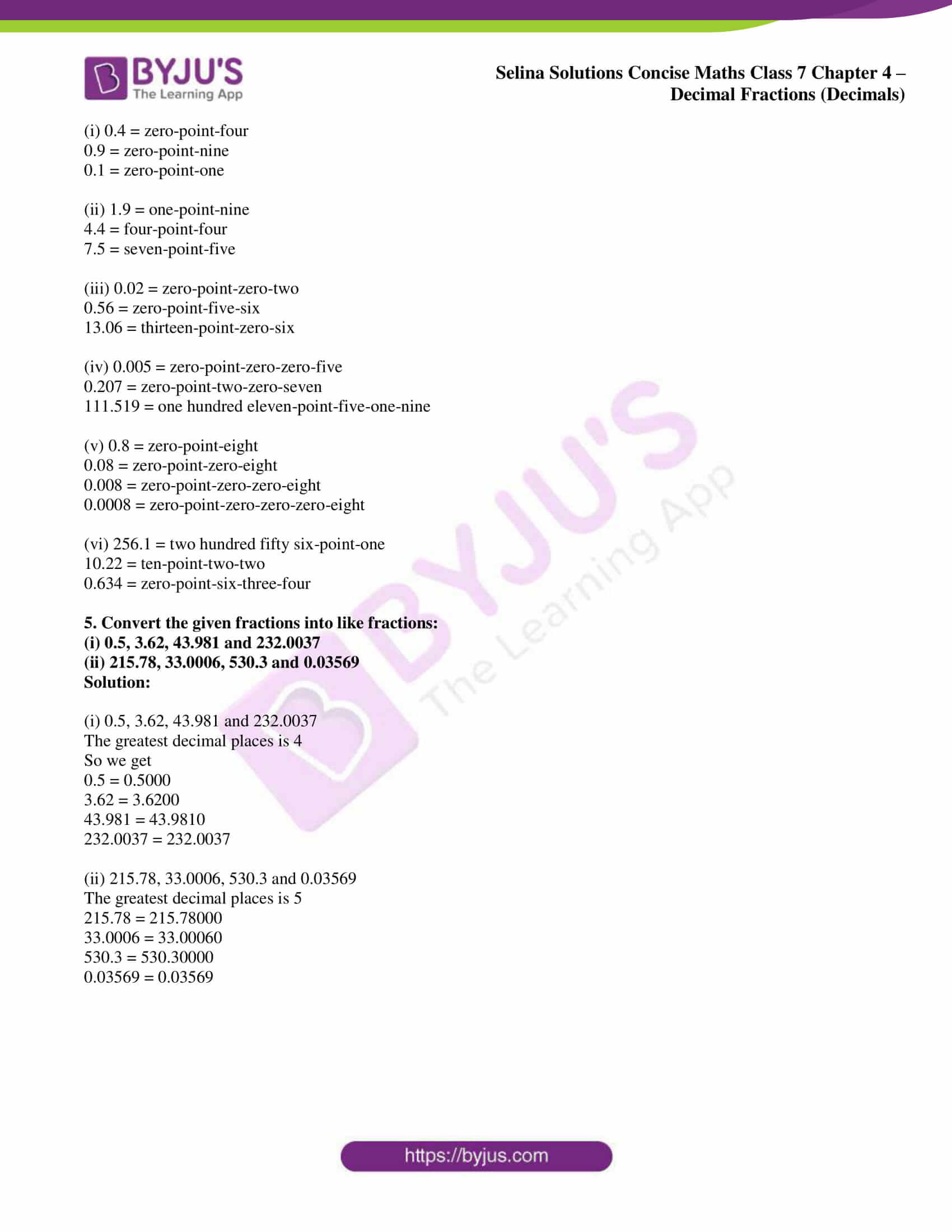### Access Selina Solutions Concise Maths Class 7 Chapter 4: Decimal Fractions (Decimals) Exercise 4A

1. Convert the following into fractions in their lowest terms:

(i) 3.75

(ii) 0.5

(iii) 2.04

(iv) 0.65

(v) 2.405

(vi) 0.085

(vii) 8.025

Solution:

(i) 3.75

We can write it as

= 375/ 100

Here the HCF of 375 and 100 is 25

= (375 ÷ 25)/ (100 ÷ 25)

So we get

= 15/4

(ii) 0.5

We can write it as

= 5/10

= 1/2

(iii) 2.04

We can write it as

= 204/100

Here the HCF of 204 and 100 is 4

= (204 ÷ 4)/ (100 ÷ 4)

So we get

= 51/25

(iv) 0.65

We can write it as

= 65/100

Here the HCF of 65 and 100 is 5

= (65 ÷ 5)/ (100 ÷ 5)

So we get

= 13/20

(v) 2.405

We can write it as

= 2405/1000

Here the HCF of 2405 and 1000 is 5

= (2405 ÷ 5)/ (1000 ÷ 5)

So we get

= 481/200

(vi) 0.085

We can write it as

= 85/1000

Here the HCF of 85 and 1000 is 5

= (85 ÷ 5)/ (1000 ÷ 5)

So we get

= 17/200

(vii) 8.025

We can write it as

= 8025/1000

Here the HCF of 8025 and 1000 is 25

= (8025 ÷ 25)/ (1000 ÷ 25)

So we get

= 321/ 40

2. Convert into decimal fractions:

(i) 2 4/5

(ii) 79/100

(iii) 37/10,000

(iv) 7543/104

(v) 3/4

(vi) 9 3/5

(vii) 8 5/8

(viii) 5 7/8

Solution:

(i) 2 4/5

We can write it as

= 14/5 × 2/2

So we get

= 28/10

= 2.8

(ii) 79/100

We can write it as

= 79/100

= 0.79

(iii) 37/10,000

We can write it as

= 37/10,000

= 0.0037

(iv) 7543/104

We can write it as

= 7543/10000

= 0.7543

(v) 3/4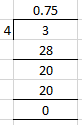By division we get

= 0.75

(vi) 9 3/5

We can write it as

= 48/5

By division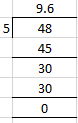So we get

= 9.6

(vii) 8 5/8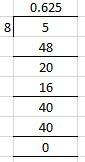By division we get

= 8.625

(viii) 5 7/8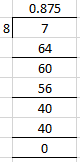By division we get

= 5.875

3. Write the number of decimal places in:

(i) 0.4762

(ii) 7.00349

(iii) 8235.403

(iv) 35.4

(v) 2.608

(vi) 0.000879

Solution:

(i) 0.4762

There are four decimal places.

(ii) 7.00349

There are five decimal places.

(iii) 8235.403

There are three decimal places.

(iv) 35.4

There is one decimal place.

(v) 2.608

There are three decimal places.

(vi) 0.000879

There are six decimal places.

4. Write the following decimals as word statements:

(i) 0.4, 0.9, 0.1

(ii) 1.9, 4.4, 7.5

(iii) 0.02, 0.56, 13.06

(iv) 0.005, 0.207, 111.519

(v) 0.8, 0.08, 0.008, 0.0008

(vi) 256.1, 10.22, 0.634

Solution:

(i) 0.4 = zero-point-four

0.9 = zero-point-nine

0.1 = zero-point-one

(ii) 1.9 = one-point-nine

4.4 = four-point-four

7.5 = seven-point-five

(iii) 0.02 = zero-point-zero-two

0.56 = zero-point-five-six

13.06 = thirteen-point-zero-six

(iv) 0.005 = zero-point-zero-zero-five

0.207 = zero-point-two-zero-seven

111.519 = one hundred eleven-point-five-one-nine

(v) 0.8 = zero-point-eight

0.08 = zero-point-zero-eight

0.008 = zero-point-zero-zero-eight

0.0008 = zero-point-zero-zero-zero-eight

(vi) 256.1 = two hundred fifty six-point-one

10.22 = ten-point-two-two

0.634 = zero-point-six-three-four

5. Convert the given fractions into like fractions:

(i) 0.5, 3.62, 43.981 and 232.0037

(ii) 215.78, 33.0006, 530.3 and 0.03569

Solution:

(i) 0.5, 3.62, 43.981 and 232.0037

The greatest decimal places is 4

So we get

0.5 = 0.5000

3.62 = 3.6200

43.981 = 43.9810

232.0037 = 232.0037

(ii) 215.78, 33.0006, 530.3 and 0.03569

The greatest decimal places is 5

215.78 = 215.78000

33.0006 = 33.00060

530.3 = 530.30000

0.03569 = 0.03569